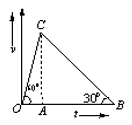The velocity-time graph of a body is shown in figure. The ratio of the …….during the intervals OA and AB is….

# The velocity-time graph of a body is shown in figure. The ratio of the .......during the intervals OA and AB is….1. A

Average velocities;1

2. B

$\frac{\mathrm{OA}}{\mathrm{OB}};\frac{1}{4}$

3. C

Average accelerations; same as distances covered

4. D

Distances covered, $\frac{1}{2}$

Register to Get Free Mock Test and Study Material

+91

Verify OTP Code (required)

### Solution:

${\overline{\mathrm{V}}}_{\mathrm{OC}}=\frac{\frac{1}{2}×\mathrm{OA}×\mathrm{AC}}{\mathrm{OA}}=\frac{\mathrm{AC}}{2}$${\overline{\mathrm{V}}}_{\mathrm{CB}}=\frac{\frac{1}{2}×\mathrm{AB}×\mathrm{AC}}{\mathrm{AB}}=\frac{\mathrm{AC}}{2}$Register to Get Free Mock Test and Study Material

+91

Verify OTP Code (required)